Next: Refrigerators Up: Classical thermodynamics Previous: The adiabatic atmosphere

## Heat engines

Thermodynamics was invented, almost by accident, in 1825 by a young French engineer called Sadi Carnot who was investigating the theoretical limitations on the efficiency of steam engines. Although we are not particularly interested in steam engines, nowadays, it is still highly instructive to review some of Carnot's arguments. We know, by observation, that it is possible to do mechanical workupon a device, and then to extract an equivalent amount of heat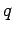, which goes to increase the internal energy of some heat reservoir. (Here, we use small lettersandto denote intrinsically positive amounts of work and heat, respectively.) An example of this is Joule's classic experiment by which he verified the first law of thermodynamics: a paddle wheel is spun in a liquid by a falling weight, and the work done by the weight on the wheel is converted into heat, and absorbed by the liquid. Carnot's question was this: is it possible to reverse this process and build a device, called a heat engine, which extracts heat energy from a reservoir and converts it into useful macroscopic work? For instance, is it possible to extract heat from the ocean and use it to run an electric generator?

There are a few caveats to Carnot's question. First of all, the work should not be done at the expense of the heat engine itself, otherwise the conversion of heat into work could not continue indefinitely. We can ensure that this is the case if the heat engine performs some sort of cycle, by which it periodically returns to the same macrostate, but, in the meantime, has extracted heat from the reservoir and done an equivalent amount of useful work. Furthermore, a cyclic process seems reasonable because we know that both steam engines and internal combustion engines perform continuous cycles. The second caveat is that the work done by the heat engine should be such as to change a single parameter of some external device (e.g., by lifting a weight) without doing it at the expense of affecting the other degrees of freedom, or the entropy, of that device. For instance, if we are extracting heat from the ocean to generate electricity, we want to spin the shaft of the electrical generator without increasing the generator's entropy; i.e., causing the generator to heat up or fall to bits.

Let us examine the feasibility of a heat engine using the laws of thermodynamics. Suppose that a heat engineperforms a single cycle. Sincehas returned to its initial macrostate, its internal energy is unchanged, and the first law of thermodynamics tell us that the work done by the enginemust equal the heat extracted from the reservoir, so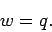(341)

The above condition is certainly a necessary condition for a feasible heat engine, but is it also a sufficient condition? In other words, does every device which satisfies this condition actually work? Let us think a little more carefully about what we are actually expecting a heat engine to do. We want to construct a device which will extract energy from a heat reservoir, where it is randomly distributed over very many degrees of freedom, and convert it into energy distributed over a single degree of freedom associated with some parameter of an external device. Once we have expressed the problem in these terms, it is fairly obvious that what we are really asking for is a spontaneous transition from a probable to an improbable state, which we know is forbidden by the second law of thermodynamics. So, unfortunately, we cannot run an electric generator off heat extracted from the ocean, because it is like asking all of the molecules in the ocean, which are jiggling about every which way, to all suddenly jig in the same direction, so as to exert a force on some lever, say, which can then be converted into a torque on the generator shaft. We know from our investigation of statistical thermodynamics that such a process is possible, in principle, but is fantastically improbable.

The improbability of the scenario just outlined is summed up in the second law of thermodynamics. This says that the total entropy of an isolated system can never spontaneously decrease, so(342)

For the case of a heat engine, the isolated system consists of the engine, the reservoir from which it extracts heat, and the outside device upon which it does work. The engine itself returns periodically to the same state, so its entropy is clearly unchanged after each cycle. We have already specified that there is no change in the entropy of the external device upon which the work is done. On the other hand, the entropy change per cycle of the heat reservoir, which is at absolute temperature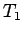, say, is given by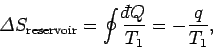(343)

whereis the infinitesimal heat absorbed by the reservoir, and the integral is taken over a whole cycle of the heat engine. The integral can be converted into the expression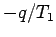because the amount of heat extracted by the engine is assumed to be too small to modify the temperature of the reservoir (this is the definition of a heat reservoir), so thatis a constant during the cycle. The second law of thermodynamics clearly reduces to(344)

or, making use of the first law of thermodynamics,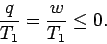(345)

Since we wish the workdone by the engine to be positive, the above relation clearly cannot be satisfied, which proves that an engine which converts heat directly into work is thermodynamically impossible.

A perpetual motion device, which continuously executes a cycle without extracting heat from, or doing work on, its surroundings, is just about possible according to Eq. (345). In fact, such a device corresponds to the equality sign in Eq. (342), which means that it must be completely reversible. In reality, there is no such thing as a completely reversible engine. All engines, even the most efficient, have frictional losses which make them, at least, slightly irreversible. Thus, the equality sign in Eq. (342) corresponds to an asymptotic limit which reality can closely approach, but never quite attain. It follows that a perpetual motion device is thermodynamically impossible. Nevertheless, the U.S. patent office receives about 100 patent applications a year regarding perpetual motion devices. The British patent office, being slightly less open-minded that its American counterpart, refuses to entertain such applications on the basis that perpetual motion devices are forbidden by the second law of thermodynamics.

According to Eq. (345), there is no thermodynamic objection to a heat engine which runs backwards, and converts work directly into heat. This is not surprising, since we know that this is essentially what frictional forces do. Clearly, we have, here, another example of a natural process which is fundamentally irreversible according to the second law of thermodynamics. In fact, the statement

It is impossible to construct a perfect heat engine which converts heat directly into work
is called Kelvin's formulation of the second law.

We have demonstrated that a perfect heat engine, which converts heat directly into work, is impossible. But, there must be some way of obtaining useful work from heat energy, otherwise steam engines would not operate. Well, the reason that our previous scheme did not work was that it decreased the entropy of a heat reservoir, at some temperature, by extracting an amount of heatper cycle, without any compensating increase in the entropy of anything else, so the second law of thermodynamics was violated. How can we remedy this situation? We still want the heat engine itself to perform periodic cycles (so, by definition, its entropy cannot increase over a cycle), and we also do not want to increase the entropy of the external device upon which the work is done. Our only other option is to increase the entropy of some other body. In Carnot's analysis, this other body is a second heat reservoir at temperature. We can increase the entropy of the second reservoir by dumping some of the heat we extracted from the first reservoir into it. Suppose that the heat per cycle we extract from the first reservoir is, and the heat per cycle we reject into the second reservoir is. Let the work done on the external device beper cycle. The first law of thermodynamics tells us that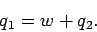(346)

Note that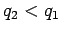if positive (i.e., useful) work is done on the external device. The total entropy change per cycle is due to the heat extracted from the first reservoir and the heat dumped into the second, and has to be positive (or zero) according to the second law of thermodynamics. So,(347)

We can combine the previous two equations to give(348)

or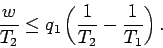(349)

It is clear that the engine is only going to perform useful work (i.e.,is only going to be positive) if. So, the second reservoir has to be colder than the first if the heat dumped into the former is to increase the entropy of the Universe more than the heat extracted from the latter decreases it. It is useful to define the efficiencyof a heat engine. This is the ratio of the work done per cycle on the external device to the heat energy absorbed per cycle from the first reservoir. The efficiency of a perfect heat engine is unity, but we have already shown that such an engine is impossible. What is the efficiency of a realizable engine? It is clear from the previous equation that(350)

Note that the efficiency is always less than unity. A real engine must always reject some energy into the second heat reservoir in order to satisfy the second law of thermodynamics, so less energy is available to do external work, and the efficiency of the engine is reduced. The equality sign in the above expression corresponds to a completely reversible heat engine (i.e., one which is quasi-static). It is clear that real engines, which are always irreversible to some extent, are less efficient than reversible engines. Furthermore, all reversible engines which operate between the two temperaturesandmust have the same efficiency,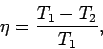(351)

irrespective of the way in which they operate.

Let us consider how we might construct one of these reversible heat engines. Suppose that we have some gas in a cylinder equipped with a frictionless piston. The gas is not necessarily a perfect gas. Suppose that we also have two heat reservoirs at temperaturesand(where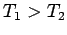). These reservoirs might take the form of large water baths. Let us start off with the gas in thermal contact with the first reservoir. We now pull the piston out very slowly so that heat energy flows reversibly into the gas from the reservoir. Let us now thermally isolate the gas and slowly pull out the piston some more. During this adiabatic process the temperature of the gas falls (since there is no longer any heat flowing into it to compensate for the work it does on the piston). Let us continue this process until the temperature of the gas falls to. We now place the gas in thermal contact with the second reservoir and slowly push the piston in. During this isothermal process heat flows out of the gas into the reservoir. We next thermally isolate the gas a second time and slowly compress it some more. In this process the temperature of the gas increases. We stop the compression when the temperature reaches. If we carry out each step properly we can return the gas to its initial state and then repeat the cycle ad infinitum. We now have a set of reversible processes by which a quantity of heat is extracted from the first reservoir and a quantity of heat is dumped into the second. We can best evaluate the work done by the system during each cycle by plotting out the locus of the gas in a-diagram. The locus takes the form of a closed curve--see Fig. 1. The net work done per cycle is the area'' contained inside this curve, since[ifis plotted vertically andhorizontally, then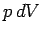is clearly an element of area under the curve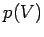]. The engine we have just described is called a Carnot engine, and is the simplest conceivable device capable of converting heat energy into useful work.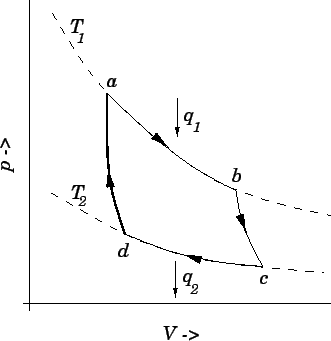For the specific case of an ideal gas, we can actually calculate the work done per cycle, and, thereby, verify Eq. (351). Consider the isothermal expansion phase of the gas. For an ideal gas, the internal energy is a function of the temperature alone. The temperature does not change during isothermal expansion, so the internal energy remains constant, and the net heat absorbed by the gas must equal the work it does on the piston. Thus,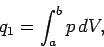(352)

where the expansion takes the gas from stateto state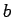. Since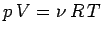, for an ideal gas, we have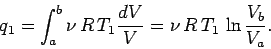(353)

Likewise, during the isothermal compression phase, in which the gas goes from stateto state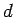, the net heat rejected to the second reservoir is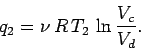(354)

Now, during adiabatic expansion or compression(355)

It follows that during the adiabatic expansion phase, which takes the gas from stateto state,(356)

Likewise, during the adiabatic compression phase, which takes the gas from stateto state,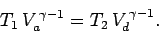(357)

If we take the ratio of the previous two equations we obtain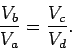(358)

Hence, the work done by the engine, which we can calculate using the first law of thermodynamics,(359)

is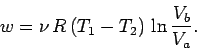(360)

Thus, the efficiency of the engine is(361)

which, not surprisingly, is exactly the same as Eq. (351).

The engine described above is very idealized. Of course, real engines are far more complicated than this. Nevertheless, the maximum efficiency of an ideal heat engine places severe constraints on real engines. Conventional power stations have many different front ends'' (e.g., coal fired furnaces, oil fired furnaces, nuclear reactors), but their back ends'' are all very similar, and consist of a steam driven turbine connected to an electric generator. The front end'' heats water extracted from a local river and turns it into steam, which is then used to drive the turbine, and, hence, to generate electricity. Finally, the steam is sent through a heat exchanger so that it can heat up the incoming river water, which means that the incoming water does not have to be heated so much by the front end.'' At this stage, some heat is rejected to the environment, usually as clouds of steam escaping from the top of cooling towers. We can see that a power station possesses many of the same features as our idealized heat engine. There is a cycle which operates between two temperatures. The upper temperature is the temperature to which the steam is heated by the front end,'' and the lower temperature is the temperature of the environment into which heat is rejected. Suppose that the steam is only heated to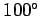C (orK), and the temperature of the environment is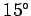C (or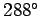K). It follows from Eq. (350) that the maximum possible efficiency of the steam cycle is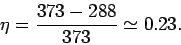(362)

So, at least 77% of the heat energy generated by the front end'' goes straight up the cooling towers! Not be surprisingly, commercial power stations do not operate withC steam. The only way in which the thermodynamic efficiency of the steam cycle can be raised to an acceptable level is to use very hot steam (clearly, we cannot refrigerate the environment). Using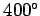C steam, which is not uncommon, the maximum efficiency becomes(363)

which is more reasonable. In fact, the steam cycles of modern power stations are so well designed that they come surprisingly close to their maximum thermodynamic efficiencies.Next: Refrigerators Up: Classical thermodynamics Previous: The adiabatic atmosphere
Richard Fitzpatrick 2006-02-02